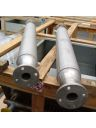# Heat exchanger calculationThe calculation of a heat exchanger, is all in all a fairly simple exercise. It involves applying a mathematical formula using a set of pre-established data and coefficients.

Obviously this is assuming we limit ourselves to the calculation of the exchange surface which is required for a certain type of thermal work.

A series of factors come into place to establish the actual size of a heat exchanger

• construction type
• limited project conditions
• internal heat flow
• turbulence
• etc…

These factors determine the actual size of the exchanger and as a result they become the essence of the project.

It is not my intention here to dwell upon complex issues but I do wish to summarize some fundamental concepts regarding heat and its transmission to the basic dimensions of a heat exchanger.

The following are some excerpts from the document that can be easily downloaded.HEAT TRANSMISSION

IN GENERAL

When there are two bodies at different temperatures the temperature of the warmer body diminishes while the temperature of the colder bodies increases. The progressive reduction of the difference in temperature must be transferred to an energy exchanger. The exchange must continue so long as there is a difference in temperature, in other words until the thermal equilibrium is reached. When the transfer of energy takes place as a result of a difference in temperature and no work is carried out on the substance, it is considered a science that is called heat transmission. The transmission of heat is essentially energy that is transmitted as a result of a difference (gradient) of temperature ∆T. This energy transfer is expressed as the amount of heat q transmitted in the unit of time t; it is a flow of heat which is named the thermal flow Q = q / t and is measured in W, since 1 J/s is equivalent to 1 W, Q is therefore the heat power. The energy transfer is carried out in three ways:
• conduction: when the transfer of heat, which is produced by the temperature gradient, takes place in a solid body or in a fluid at rest;
• convection: on the other hand is the heat transfer that takes place between a surface and a fluid in motion with different temperatures;
• irradiation: all surfaces that are at a given temperature emit energy in the form of electromagnetic waves. Therefore, in the absence of a medium located between them, the heat between the two surfaces at different temperatures is transferred only via irradiation.
These forms of transmission almost always coexist.

QUANTITY OF HEAT

The amount of heat, contained in a liquid gas, or in a body, is usually:

q = cp• m •∆T

where:

• q= the amount of heat, in J
• cp= the heat capacity mass at constant pressure, in J/(kg •K)
• m= the body mass, in kg∆T= the difference in temperature, in K

…………………………………………………….

EXCHANGER PROJECT

In order to design a heat exchanger we must correlate the amount of heat transmitted per unit of time Q with the input and output temperatures of the two fluids of area A of the total surface required for the given heat exchange.

Using the energy balance equation of hot fluid open systems (subscript c) and cold fluid open systems (subscript f), characterized by the mass flow rate mc and mf, you obtain the following two expressions.
The energy balance equation, written in terms of heat flow and through the output enthalpies (subscript 2) and input enthalpies (subscript 1), of the two fluids is:
Q = m ( h2 – h1)
Keeping in mind that, a perfect gas enthalpy expressed as a function of the temperature is:
h = c p • T

we have a first equation relating to the cold fluid, ie the flow Q that enters the cold fluid, thus increasing the temperature, is:
Q = mf • c pf • ( t f2 – t f1) (1)
c pf being the thermal capacity mass of the cold fluid.
Removing the heat flow Q however, decreases the temperature of the hot fluid from the input value t c1 to the output value tc 2, the equation for the heat flow is therefore:
Q = mc • c pc• ( t c1 – t c 2)
c pc being the thermal capacity mass of the hot fluid.
Once the thermal gradient is known, or calculated based the above-mentioned balance equations, we proceed to the sizing of the area A of the exchange surface, using an equation which links the heat transmitted in the unit of time Q between the two
fluids with area A and the average difference in temperature ΔTm of the two fluids.

Q =U ·A ·ΔTm
The link between the thermal flow Q and the product of area A for the average difference in temperature is expressed by the overall coefficient of exchange U, which is determined empirically.

Heat exchanger calculation